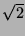Next: Flow Of Control Features Up: Language Features Previous: Implementation Features

Numeric Features

To some degree, the reason for the existence of Nickle is the support for infinite-precision integer and rational arithmetic. By default, non-integer quantities are represented as rationals, which ensures that precision will never be lost by the computation. For convenient comparison and for familiarity's sake, rationals behave syntactically like floating-point numbers: they are input and output using a floating decimal point.

To avoid loss of precision due to decimal conversion, both output and input representations of rational numbers support repeating decimals''. For example, the constant 0.1{6} is the Nickle decimal representation of. Using sophisticated techniques based on a factored representation, Nickle is capable of calculating the minimum-length repeat for the decimal representation of an arbitrary rational number. This can be very expensive in some cases, however, so by default repeating representations beyond a certain number of digits will be truncated. While this may lead to loss of precision on input or output, it can be disabled, and in any case all calculations will still be performed internally to full precision.

Some real numbers (irrational numbers such asand transcendental numbers such as) are not precisely representable as rational numbers. In order to perform calculations involving these quantities, Nickle provides its own implementation of floating-point arithmetic with user-settable mantissa precision and infinite precision exponents. A sensible numeric type hierarchy and well-defined rules for combining various precisions means that Nickle is generally very good at retaining precision in all numeric calculations.Next: Flow Of Control Features Up: Language Features Previous: Implementation Features
Bart Massey 2001-04-19